## Error Detection in Computer Networks-

 Error detection is a technique that is used to check if any error occurred in the data during the transmission.

Some popular error detection methods are-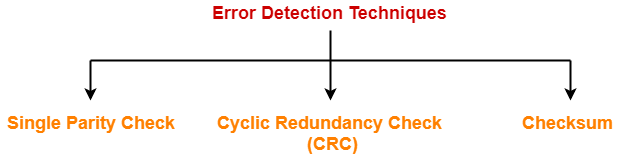1. Single Parity Check
2. Cyclic Redundancy Check (CRC)
3. Checksum

## Checksum-

Checksum is an error detection method.

Error detection using checksum method involves the following steps-

## Step-01:

At sender side,

• If m bit checksum is used, the data unit to be transmitted is divided into segments of m bits.
• All the m bit segments are added.
• The result of the sum is then complemented using 1’s complement arithmetic.
• The value so obtained is called as checksum.

## Step-02:

• The data along with the checksum value is transmitted to the receiver.

## Step-03:

• If m bit checksum is being used, the received data unit is divided into segments of m bits.
• All the m bit segments are added along with the checksum value.
• The value so obtained is complemented and the result is checked.

Then, following two cases are possible-

### Case-01: Result = 0

If the result is zero,

• Receiver assumes that no error occurred in the data during the transmission.

### Case-02: Result ≠ 0

If the result is non-zero,

• Receiver assumes that error occurred in the data during the transmission.

## Checksum Example-

Consider the data unit to be transmitted is-

10011001111000100010010010000100

Consider 8 bit checksum is used.

### Step-01:

At sender side,

The given data unit is divided into segments of 8 bits as-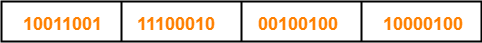Now, all the segments are added and the result is obtained as-

• 10011001 + 11100010 + 00100100 + 10000100 = 1000100011
• Since the result consists of 10 bits, so extra 2 bits are wrapped around.
• 00100011 + 10 = 00100101 (8 bits)
• Now, 1’s complement is taken which is 11011010.
• Thus, checksum value = 11011010

### Step-02:

• The data along with the checksum value is transmitted to the receiver.

### Step-03:

• The received data unit is divided into segments of 8 bits.
• All the segments along with the checksum value are added.
• Sum of all segments + Checksum value = 00100101 + 11011010 = 11111111
• Complemented value = 00000000
• Since the result is 0, receiver assumes no error occurred in the data and therefore accepts it.

## Important Notes-

### Note-01:

• Consider while adding the m bit segments, the result obtained consists of more than m bits.
• Then, wrap around the extra bits and add to the result so that checksum value consists of m bits.

### Note-02:

• While calculating the checksum, if checksum value is needed, then assume it to be zero.
• After calculating the checksum value, substitute the checksum value in the checksum field.

### Note-03:

• The checksum is used in the internet by several protocols although not at the data link layer.

Also Read- Cyclic Redundancy Check (CRC)

## Problem-

Checksum value of 1001001110010011 and 1001100001001101 of 16 bit segment is-

1. 1010101000011111
2. 1011111000100101
3. 1101010000011110
4. 1101010000111111

## Solution-

We apply the above discussed algorithm to calculate the checksum.

• 1001001110010011 + 1001100001001101 = 10010101111100000
• Since, the result consists of 17 bits, so 1 bit is wrapped around and added to the result.
• 0010101111100000 + 1 = 0010101111100001
• Now, result consists of 16 bits.
• Now, 1’s complement is taken which is 1101010000011110
• Thus, checksum value = 1101010000011110

Thus, Option (C) is correct.

To gain better understanding about Checksum Method,

Watch this Video Lecture

Next Article- Access Control Methods | Introduction

Get more notes and other study material of Computer Networks.

Watch video lectures by visiting our YouTube channel LearnVidFun.

## Error Detection in Computer Networks-

When sender transmits data to the receiver, the data might get scrambled by noise or data might get corrupted during the transmission.

 Error detection is a technique that is used to check if any error occurred in the data during the transmission.

## Error Detection Methods-

Some popular error detection methods are-1. Single Parity Check
2. Cyclic Redundancy Check (CRC)
3. Checksum

## Single Parity Check-

In this technique,

• One extra bit called as parity bit is sent along with the original data bits.
• Parity bit helps to check if any error occurred in the data during the transmission.

## Steps Involved-

Error detection using single parity check involves the following steps-

## Step-01:

At sender side,

• Total number of 1’s in the data unit to be transmitted is counted.
• The total number of 1’s in the data unit is made even in case of even parity.
• The total number of 1’s in the data unit is made odd in case of odd parity.
• This is done by adding an extra bit called as parity bit.

## Step-02:

• The newly formed code word (Original data + parity bit) is transmitted to the receiver.

## Step-03:

• The total number of 1’s in the received code word is counted.

Then, following cases are possible-

• If total number of 1’s is even and even parity is used, then receiver assumes that no error occurred.
• If total number of 1’s is even and odd parity is used, then receiver assumes that error occurred.
• If total number of 1’s is odd and odd parity is used, then receiver assumes that no error occurred.
• If total number of 1’s is odd and even parity is used, then receiver assumes that error occurred.

## Parity Check Example-

Consider the data unit to be transmitted is 1001001 and even parity is used.

Then,

### At Sender Side-

• Total number of 1’s in the data unit is counted.
• Total number of 1’s in the data unit = 3.
• Clearly, even parity is used and total number of 1’s is odd.
• So, parity bit = 1 is added to the data unit to make total number of 1’s even.
• Then, the code word 10010011 is transmitted to the receiver.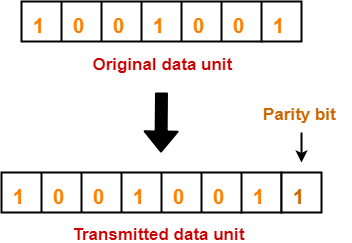• After receiving the code word, total number of 1’s in the code word is counted.
• Even parity is used and total number of 1’s is even.
• So, receiver assumes that no error occurred in the data during the transmission.

• This technique is guaranteed to detect an odd number of bit errors (one, three, five and so on).
• If odd number of bits flip during transmission, then receiver can detect by counting the number of 1’s.

## Limitation-

• This technique can not detect an even number of bit errors (two, four, six and so on).
• If even number of bits flip during transmission, then receiver can not catch the error.

### EXAMPLE

• Consider the data unit to be transmitted is 10010001 and even parity is used.
• Then, code word transmitted to the receiver = 100100011
• Consider during transmission, code word modifies as 101100111. (2 bits flip)
• On receiving the modified code word, receiver finds the number of 1’s is even and even parity is used.
• So, receiver assumes that no error occurred in the data during transmission though the data is corrupted.

To gain better understanding about single parity check,

Watch this Video Lecture

Next Article- Cyclic Redundancy Check

Get more notes and other study material of Computer Networks.

Watch video lectures by visiting our YouTube channel LearnVidFun.

## Error Detection in Computer Networks-

 Error detection is a technique that is used to check if any error occurred in the data during the transmission.

Some popular error detection methods are-1. Single Parity Check
2. Cyclic Redundancy Check (CRC)
3. Checksum

## Cyclic Redundancy Check-

• Cyclic Redundancy Check (CRC) is an error detection method.
• It is based on binary division.

## CRC Generator-

• CRC generator is an algebraic polynomial represented as a bit pattern.
• Bit pattern is obtained from the CRC generator using the following rule-

 The power of each term gives the position of the bit and the coefficient gives the value of the bit.

## Example-

Consider the CRC generator is x7 + x6 + x4 + x3 + x + 1.

The corresponding binary pattern is obtained as-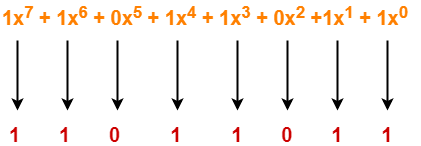Thus, for the given CRC generator, the corresponding binary pattern is 11011011.

## Properties Of CRC Generator-

The algebraic polynomial chosen as a CRC generator should have at least the following properties-

### Rule-01:

• It should not be divisible by x.
• This condition guarantees that all the burst errors of length equal to the length of polynomial are detected.

### Rule-02:

• It should be divisible by x+1.
• This condition guarantees that all the burst errors affecting an odd number of bits are detected.

## Important Notes-

If the CRC generator is chosen according to the above rules, then-

• CRC can detect all single-bit errors
• CRC can detect all double-bit errors provided the divisor contains at least three logic 1’s.
• CRC can detect any odd number of errors provided the divisor is a factor of x+1.
• CRC can detect all burst error of length less than the degree of the polynomial.
• CRC can detect most of the larger burst errors with a high probability.

## Steps Involved-

Error detection using CRC technique involves the following steps-

### Step-01: Calculation Of CRC At Sender Side-

At sender side,

• A string of n 0’s is appended to the data unit to be transmitted.
• Here, n is one less than the number of bits in CRC generator.
• Binary division is performed of the resultant string with the CRC generator.
• After division, the remainder so obtained is called as CRC.
• It may be noted that CRC also consists of n bits.

### Step-02: Appending CRC To Data Unit-

At sender side,

• The CRC is obtained after the binary division.
• The string of n 0’s appended to the data unit earlier is replaced by the CRC remainder.

• The newly formed code word (Original data + CRC) is transmitted to the receiver.

### Step-04: Checking at Receiver Side-

• The transmitted code word is received.
• The received code word is divided with the same CRC generator.
• On division, the remainder so obtained is checked.

The following two cases are possible-

### Case-01: Remainder = 0

If the remainder is zero,

• Receiver assumes that no error occurred in the data during the transmission.

### Case-02: Remainder ≠ 0

If the remainder is non-zero,

• Receiver assumes that some error occurred in the data during the transmission.

## Problem-01:

A bit stream 1101011011 is transmitted using the standard CRC method. The generator polynomial is x4+x+1. What is the actual bit string transmitted?

## Solution-

• The generator polynomial G(x) = x4 + x + 1 is encoded as 10011.
• Clearly, the generator polynomial consists of 5 bits.
• So, a string of 4 zeroes is appended to the bit stream to be transmitted.
• The resulting bit stream is 11010110110000.

Now, the binary division is performed as-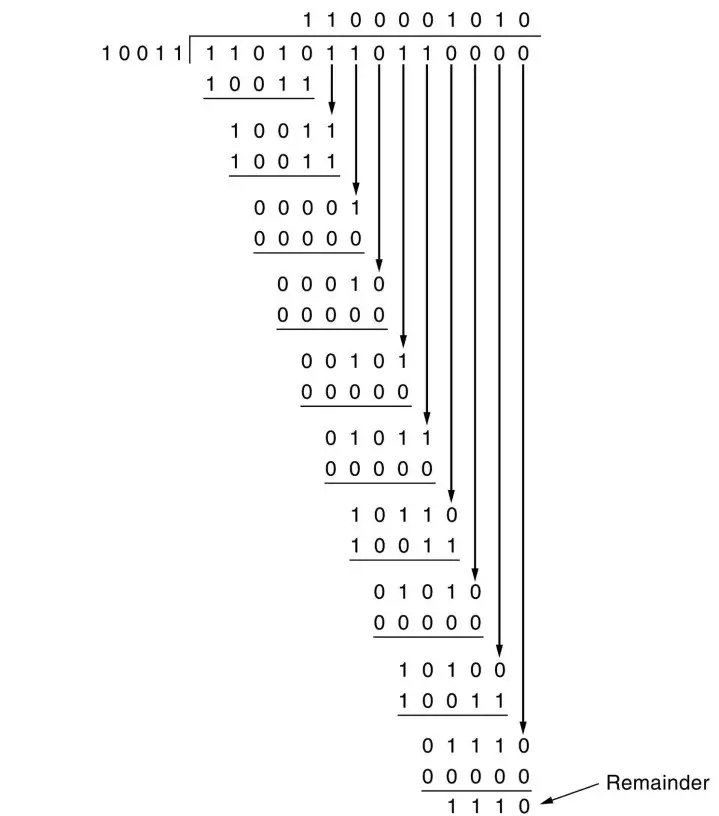From here, CRC = 1110.

Now,

• The code word to be transmitted is obtained by replacing the last 4 zeroes of 11010110110000 with the CRC.
• Thus, the code word transmitted to the receiver = 11010110111110.

## Problem-02:

A bit stream 10011101 is transmitted using the standard CRC method. The generator polynomial is x3+1.

1. What is the actual bit string transmitted?
2. Suppose the third bit from the left is inverted during transmission. How will receiver detect this error?

## Solution-

### Part-01:

• The generator polynomial G(x) = x3 + 1 is encoded as 1001.
• Clearly, the generator polynomial consists of 4 bits.
• So, a string of 3 zeroes is appended to the bit stream to be transmitted.
• The resulting bit stream is 10011101000.

Now, the binary division is performed as-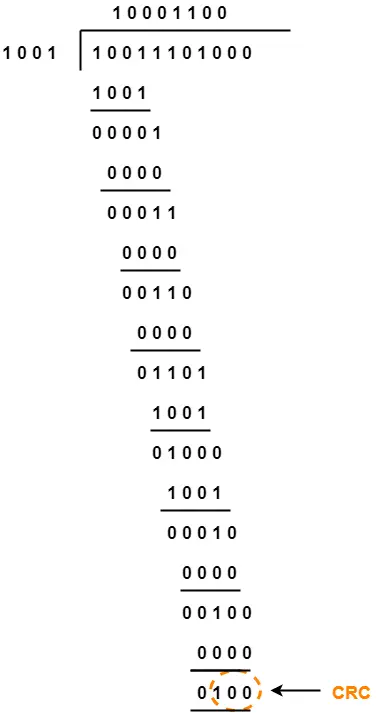From here, CRC = 100.

Now,

• The code word to be transmitted is obtained by replacing the last 3 zeroes of 10011101000 with the CRC.
• Thus, the code word transmitted to the receiver = 10011101100.

## Part-02:

According to the question,

• Third bit from the left gets inverted during transmission.

Now,

• Receiver performs the binary division with the same generator polynomial as-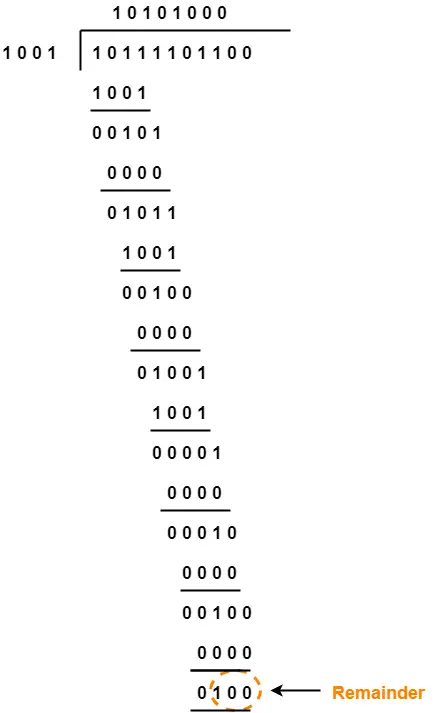From here,

• The remainder obtained on division is a non-zero value.
• This indicates to the receiver that an error occurred in the data during the transmission.
• Therefore, receiver rejects the data and asks the sender for retransmission.

To watch video solution, click here.

To gain better understanding about Cyclic Redundancy Check,

Watch this Video Lecture

Next Article- Checksum

Get more notes and other study material of Computer Networks.

Watch video lectures by visiting our YouTube channel LearnVidFun.